Hostname: page-component-546b4f848f-bvkm5 Total loading time: 0 Render date: 2023-06-05T08:40:32.774Z Has data issue: false Feature Flags: { "useRatesEcommerce": true } hasContentIssue false

# Jeśmanowicz’ Conjecture with Congruence Relations. II

Published online by Cambridge University Press:  20 November 2018

## Abstract

HTML view is not available for this content. However, as you have access to this content, a full PDF is available via the ‘Save PDF’ action button.

Let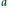$a$,$b$, and$c$ be primitive Pythagorean numbers such that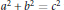${{a}^{2}}\,+\,{{b}^{2}}\,=\,{{c}^{2}}$ with$b$ even. In this paper, we show that if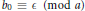${{b}_{0}}\,\equiv \,\in \,\,\,\left( \bmod \,a \right)$ with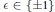$\text{ }\!\!\varepsilon\!\!\text{ }\,\in \,\left\{ \pm 1 \right\}$ for certain positive divisors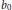${{b}_{0}}$ of$b$, then the Diophantine equation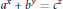${{a}^{x}}\,+\,{{b}^{y}}\,=\,{{c}^{z}}$ has only the positive solution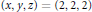$\left( x,\,y,\,z \right)\,=\,\left( 2,\,2,\,2 \right)$.

## Keywords

Type
Research Article
Information
Canadian Mathematical Bulletin , 01 September 2014 , pp. 495 - 505